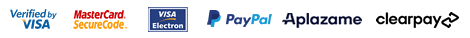▼ Language: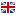English

##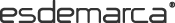WOMAN | MAN | KIDS
Favourites
My account# Buy DIM Underwear for Man

Brands
Sizes

Select
Price

Select
Colour
Select
Style

Select
Material

Select
 Order Relevance Ascending price Descending price Discount New in Delivery date Ratings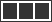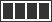### DIM Pack of 2 slips Unno by DIM Basic light blue, marine

14.00 €
 Size 5 = 56 6 = 60 Add
Sizes: 5 = 56   6 = 60

### DIM Pack of 2 slips Unno by DIM Basic blue, black

14.00 €
 Size 3 = 48 4 = 52 6 = 60 Add
Sizes: 3 = 48   4 = 52   6 = 60

### DIM Pack of 2 slips Unno by DIM Basic blue, grey

14.00 €
 Size 3 = 48 4 = 52 5 = 56 6 = 60 Add
Sizes: 3 = 48   4 = 52   5 = 56   6 = 60

20.90 €
Sizes: XXL

### DIM Pack of 2 Cotton Stretch Boxers Basic and Economical Style blue

19.90 €
 Size 2 = 44 3 = 48 4 = 52 5 = 56 Add
Sizes: 2 = 44   3 = 48   4 = 52   5 = 56

### DIM Pack of 2 Cotton Stretch Boxers Basic and Economic Style red, gray

19.90 €
 Size 3 = 48 4 = 52 5 = 56 Add
Sizes: 3 = 48   4 = 52   5 = 56

### DIM Pack of 2 white cotton t-shirts

20.90 €
Sizes: M   L   XXL

### DIM Pack of 2 cotton boxer shorts with striking blue print waistbands

20.90 €
 Size 3 = 48 4 = 52 Add
Sizes: 3 = 48   4 = 52

### DIM Pack of 3 blue, light blue, black elastic cotton boxers

39.90 €
 Size 2 = 44 3 = 48 4 = 52 5 = 56 Add
Sizes: 2 = 44   3 = 48   4 = 52   5 = 56

14.00 €
Sizes: 6 = 60

### DIM Pack of 2 slips Unno by DIM Basic white, black

14.00 €
 Size 3 = 48 5 = 56 6 = 60 Add
Sizes: 3 = 48   5 = 56   6 = 60

### DIM Pack of 2 long sport boxer shorts, ultra elastic fabric, white, grey

31.90 €
 Size 2 = 44 4 = 52 Add
Sizes: 2 = 44   4 = 52

### DIM Pack of 2 Unno by DIM Basic boxers white, black

14.00 €
 Size 3 = 48 4 = 52 5 = 56 6 = 60 Add
Sizes: 3 = 48   4 = 52   5 = 56   6 = 60

14.00 €
Sizes: 6 = 60

### DIM Pack of 2 slips Unno by DIM Basic fuchsia, black

14.00 €
 Size 3 = 48 4 = 52 5 = 56 6 = 60 Add
Sizes: 3 = 48   4 = 52   5 = 56   6 = 60

### DIM Pack of 2 black cotton boxers with coloured waistband

23.90 €
 Size 2 = 44 3 = 48 4 = 52 Add
Sizes: 2 = 44   3 = 48   4 = 52

### DIM Pack of 2 sport ultra-elastic woven boxer shorts green, black

31.90 €
 Size 4 = 52 5 = 56 Add
Sizes: 4 = 52   5 = 56

### DIM Pack of 3 light grey, dark grey, black cotton boxers

35.00 €
 Size 2 = 44 4 = 52 5 = 56 Add
Sizes: 2 = 44   4 = 52   5 = 56

### DIM Pack of 2 Unno by DIM Basic marine boxers, black

14.00 €
 Size 3 = 48 4 = 52 5 = 56 6 = 60 Add
Sizes: 3 = 48   4 = 52   5 = 56   6 = 60

14.00 €
Sizes: 6 = 60

14.00 €
Sizes: 6 = 60

26.90 €
Sizes: 2 = 44

14.00 €
Sizes: 6 = 60

### DIM Pack of 2 blue seamless boxers

31.90 €
 Size 3 = 48 4 = 52 Add
Sizes: 3 = 48   4 = 52

### DIM Pack of 2 Unno by DIM Basic boxers blue, grey

14.00 €
 Size 3 = 48 4 = 52 5 = 56 6 = 60 Add
Sizes: 3 = 48   4 = 52   5 = 56   6 = 60

### DIM Black elastic cotton sports boxer

22.00 €
 Size 3 = 48 4 = 52 6 = 60 Add
Sizes: 3 = 48   4 = 52   6 = 60

### DIM 2-pack of blue, grey motion-adjustable boxer shorts

31.90 €
 Size 3 = 48 4 = 52 6 = 60 Add
Sizes: 3 = 48   4 = 52   6 = 60

### DIM Pack of 2 Unno by DIM Basic black boxers

14.00 €
 Size 2 = 44 6 = 60 Add
Sizes: 2 = 44   6 = 60

### DIM Pack of 2 slips Unno by DIM Basic black

14.00 €
 Size 3 = 48 4 = 52 5 = 56 6 = 60 Add
Sizes: 3 = 48   4 = 52   5 = 56   6 = 60

### DIM Pack of 3 black elastic cotton boxers

30.90 €
 Size 2 = 44 3 = 48 4 = 52 Add
Sizes: 2 = 44   3 = 48   4 = 52

20.90 €
Sizes: 5 = 56

### DIM Pack of 2 black elastic cotton slips

24.50 €
 Size 2 = 44 3 = 48 Add
Sizes: 2 = 44   3 = 48

### DIM Pack of 2 black combed cotton boxers

31.90 €
 Size 3 = 48 4 = 52 5 = 56 6 = 60 Add
Sizes: 3 = 48   4 = 52   5 = 56   6 = 60

### DIM Pack of 2 sport boxers, ultra elastic fabric, white, grey

31.90 €
 Size 2 = 44 3 = 48 4 = 52 5 = 56 Add
Sizes: 2 = 44   3 = 48   4 = 52   5 = 56

### DIM Pack of 2 long sports microfibre boxers with breathable mesh grey, black

29.90 €
Sizes: 2   3   4

### DIM Pack of 3 black elastic cotton slips

26.90 €
 Size 2 = 44 3 = 48 4 = 52 5 = 56 Add
Sizes: 2 = 44   3 = 48   4 = 52   5 = 56

### DIM Pack of 2 slips Unno by DIM Basic blue, grey

14.00 €
 Size 5 = 56 6 = 60 Add
Sizes: 5 = 56   6 = 60

### DIM Pack of 2 long sport boxers, ultra elastic marine fabric, red

31.90 €
 Size 2 = 44 3 = 48 4 = 52 Add
Sizes: 2 = 44   3 = 48   4 = 52

### DIM Pack of 3 light blue, navy, blue cotton boxers

35.00 €
 Size 2 = 44 6 = 60 Add
Sizes: 2 = 44   6 = 60

### DIM Pack of 2 Unno by DIM Basic grey, black

14.00 €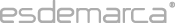Esdemarca Esdemarca Our commitments Privacy policy About cookies Your orders Help Security Shipping costs Easy return Contacts Are you a supplier? Join our team Customer service Make your suggestion Connect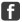Facebook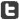Twitter Go to mobile versionEsdemarca · Copyright © Fusion Lab, S.L. 2021  |  Legal warning B66138827 · Calle Manzanares, 4, 28005, Madrid (España) · consultas@esdemarca.com · +34 91 769 72 17